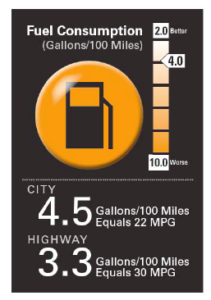# Miles Per Gallon Java Program | 4 Ways

Java Program To Calculate Miles Per Gallon – In this article, we will detail in on all the possible methods used to calculate miles per gallon in Java. The following source code has been written in multiple ways for easy understand.

Suitable examples and sample programs have been included in order to make you understand simply.

• Using Standard Method
• Using Command Line Arguments
• Using Static Method
• Using Separate ClassMiles per Gallon is considered to be the average mileage of a motor vehicle which uses Petrol or Diesel as fuel. Miles represents the distance the vehicle travelled in the units of Miles.

1 Gallon is a measure of the fuel used. To convert 1 Gallon into the much more normally used SI units,

1 Gallon = 3.785 litres.

Hence, the lower the gallons of fuel is consumed, the better is its mileage.

Thus, the various methods to calculate Miles per Gallon in Java Programming are as follows:

## Java Code Miles Per Gallon

In this method, the entire logic along with the input output operations is written in the main method of the Java Class.

For any problem, the first step is to take inputs as desired followed by the necessary steps to be taken and operations to be performed to arrive at the output.

In our present problem, we require two inputs- Miles traveled (miles) of the decimal type therefore, we make use of double and Gallons (gallons) which is also of type double. After getting the input by making use of scanner class, we make use of the following formula:

Mileage = Miles/Gallons

This is the desired output which is also of type double which can be displayed on our output screen.

Output1:

### Using Command Line Arguments

Just like making use of scanner class in the above example, there is another possibility to take inputs at run time. This is by making use of command line arguments.

In here, following the run command with the name of Java code to be executed, after a space a first argument (arg) is to be given which will be stored in a variable (km) which is again followed by a space and the second argument (arg) which will be stored in another variable (gallons or g).

While taking inputs, since our first input is in km format whereas we require it in miles, conversion is to be made. Th formula used for conversion is:

Miles (m)= km/1.609344

Now, with all the requirements in hand, the calculation is to be performed using the formula

Mileage = Miles/Gallons, which is the desired output.

Output1:

#### Using Static Method

In both the above examples, it is observed that, the entire code has been written in the main method itself which is not efficient when considering the readability factor. Hence, in order to make the readability better, it is advisable to split up the code based on its logic.

In the current problem, the main method can contain all the input output operations and call another static method (distance).

In this static method (distance), the inputs taken (miles and gallons) can be passed as arguments. The static method (distance) will then perform the calculations required using the formula,

This is then returned to the main method in which the output operations will be performed to display on the output screen.

Using Separate Class

When everything is written in a single class and a code is lengthy, it becomes a hideous task to find the portion of code we would like to view.

To make this approach easier, it is better to split the code into different classes based on their logic and functionality.

In this problem, we can split the code into two portions or classes.

One class consisting of the main method which takes care of reading the inputs (km and g). The main method then creates an object referencing the other class (MgpCal).

When object is created, the constructor in that class gets executed. This constructor consists of statements to perform operations to find the miles per gallon.

First it converts kilometres to miles and then finds the mileage (miles/gallons).

This is stored in a variable of class MgpCal which is printed in the main method using the object created for this class and thereby calling the variable with that object. This is round figured using round method of the Math package and printed.

Output:

x

## Plus Star Pattern Java Program | Patterns

Java program to print plus star pattern program – We have written the below print/draw ...